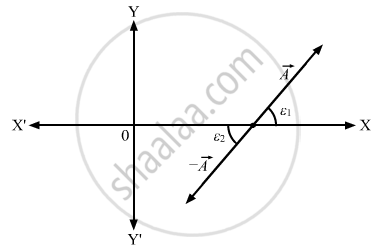Department of Pre-University Education, KarnatakaPUC Karnataka Science Class 11

# Let ε1 and ε2 Be the Angles Made by → a and → − a with the Positive X-axis. Show that Tan ε1 = Tan ε2. Thus, Giving Tan ε Does Not Uniquely Determine the Direction of → a . - Physics

Let ε1 and ε2 be the angles made by  $\vec{A}$ and -$\vec{A}$ with the positive X-axis. Show that tan ε1 = tan ε2. Thus, giving tan ε does not uniquely determine the direction of $\vec{A}$.

#### Solution

The direction of - $\vec{A}$ is opposite to $\vec{A}$.So, if vector $\vec{A}$ and $- \vec{A}$ make the angles ε1 and ε2 with the X-axis, respectively, then ε1 is equal to ε2 as shown in the figure:Here, tan ε1 = tan ε2
Because these are alternate angles.
Thus, giving tan ε does not uniquely determine the direction of $- \vec{A}$.

Concept: What is Physics?
Is there an error in this question or solution?
Chapter 2: Physics and Mathematics - Short Answers [Page 28]

#### APPEARS IN

HC Verma Class 11, Class 12 Concepts of Physics Vol. 1
Chapter 2 Physics and Mathematics
Short Answers | Q 9 | Page 28
Share